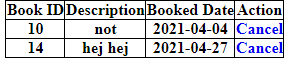# Delete a record from database using node.js/Express.js

I have been sitting with this project for 2 days and couldn’t figure out what is wrong. I Will be grateful if u could help me point out the problem

• I want to delete a record from the HTML table. I can retrieve the book_id, how can I access the book_id in my delete function.
``````<script>
fetch('http://localhost:3003/booked').then(function (response) {
return response.json();
}).then(function (data) {
appendData(data);
}).catch(function (err) {
console.log("error:" + err);
});
function appendData(data) {
let tbodyContainer = document.getElementById("tbody");
for (let i = 0; i < data.length; i++) {
let tr = document.createElement("tr");
tr.innerHTML =
"<tr>"
+
"<th>" + data[i].book_id + "</th>" +
"<th>" + data[i].descr + "</th>" +
"<th>" + data[i].book_date + "</th>" +
`<th> <a href='/booked/\${data[i].book_id}'>Cancel</a></th>` +
"</tr>";
tbodyContainer.appendChild(tr);
}
}
</script>
``````
• How can I retrieve the book_id from the HTML table and pass it to the delete function below, so that I can compare the book_id and delete that record. When I console.log the book_id, it returns `undefined`
``````app.delete("/booked/:book_id", (req, res) => {
const book_id = req.params.book_id;
console.log("--------------> " + book_id);
if (!req.session.loggedin) {
} else {
connection.query("DELETE FROM calendar.book WHERE book_id= '" + book_id + "'", function (err, result) {
if (err) {
throw err;
} else {
res.redirect("./myBookings");
}
});
}
});
``````Check `typeof book_id` because I would not be to surprised if the original `id` is an object. When you grab the id from your params you are getting a `string` but the original `id` may in fact not be a `string`. What sort of database are you working with?

I’ve finally solved the problem, and this is how I’ve solved it. After a few days of trying to figure it out, I read the documentation about req. query, and changed the delete route to
`<th> <a href='/delete?book_id=\${data[i].book_id}'>Cancel</a></th>`

``````fetch('http://localhost:3003/booked').then(function (response) {
return response.json();
}).then(function (data) {
appendData(data);
}).catch(function (err) {
console.log("error:" + err);
});
function appendData(data) {
let tbodyContainer = document.getElementById("tbody");
for (let i = 0; i < data.length; i++) {
let tr = document.createElement("tr");
tr.innerHTML =
"<tr>"
+
"<th>" + data[i].book_id + "</th>" +
"<th>" + data[i].descr + "</th>" +
"<th>" + data[i].book_date + "</th>" +
`<th> <a href='/booked/\${data[i].book_id}'>Cancel</a></th>` +
"</tr>";
tbodyContainer.appendChild(tr);
}
}
</script>
``````

And inside the delete express route, I’ve changed the
`req.params.book_id` to `req.query.book_id`, and changed the MySQL query (just removed the quotes `( ' ')` in between the book_id)
Below is the full code of the delete route.

``````app.get("/delete", (req, res) => {
const book_id = req.query.book_id;
console.log("--------------> " + book_id); //<------------------------------
if (!req.session.loggedin) {
} else {
connection.query("DELETE FROM calendar.book WHERE book_id= " + req.query.book_id, function (err, result) {
if (err) {
throw err;
} else {
res.redirect("./myBookings");
}
});
}
});
``````

NOTE - This may not be the best solution out there, I am just a newbie

1 Like

This topic was automatically closed 182 days after the last reply. New replies are no longer allowed.